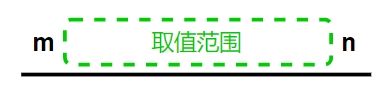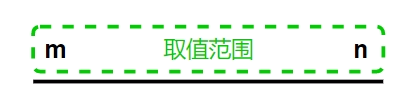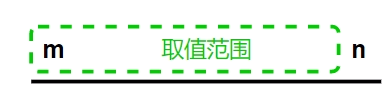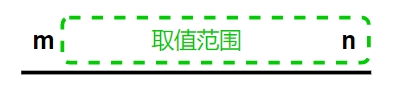• 累计撰写 45 篇文章
• 累计创建 40 个标签
• 累计收到 450 条评论

### 目 录CONTENT# JS中生成指定范围随机数2021-01-19 / 0 评论 / 5 点赞 / 2,889 阅读 / 1,206 字 / 正在检测是否收录...广告

😄在日常工作中，我们经常会需要生成一些随机数，相信大家最熟悉的就是 Math.random() 这个api，本文将介绍生成指定范围随机数的方法。

😏首先我们复习一下这几个api，后面会用到：
1、Math.random()

2、Math.floor()

3、Math.ceil()

4、Math.round()

#### 一、生成m到n之间的数字，不包含m和nconst genBetween = (m, n) => Math.round(Math.random() * (n - m - 2)) + m + 1;


#### 二、生成m到n之间的数字，包含m和nconst genBetweenAll = (m, n) => Math.floor(Math.random() * (n - m + 1)) + m;


#### 三、生成m到n之间的数字，包含m，不包含nconst genBetweenLeft = (m, n) => Math.floor(Math.random() * (n - m)) + m;


#### 四、生成m到n之间的数字，不包含m，包含nconst genBetweenRight = (m, n) => Math.floor(Math.random() * (n - m) + 1) + m;


### 参考文章

JS生成某个范围的随机数【四种情况详解】

5
1.2.广告
• 5
1.2.